# NCERT Exemplar Solutions for Class 10 Maths Chapter 8 - Introduction To Trigonometry And Its Equations

## NCERT Exemplar Solutions Class 10 Maths Chapter 8 – Free PDF Download

NCERT Exemplar Class 10 Maths Chapter 8 Trigonometry and Its Equations, provided here for students to prepare for board exams. These exemplars problems and solutions are designed by our Maths subject experts with respect to CBSE Syllabus (2021-2022). Students can extensively make use of the exemplar and have productive study time. Moreover, students will find answers to all the tough questions and they will be able to prepare well as well as deliver better performance in the exams.

NCERT Exemplar Class 10 Maths Chapter 8 mainly focuses on educating students about topics like trigonometric identities and trigonometric ratios. In this chapter, they will learn solving problems based on trigonometric ratios for specific and complementary angles and establishing identities for the trigonometric ratios. However, students have to be thorough with the concepts mentioned in the chapter to solve the problems without any difficulties. Click here to solve exemplars for all the chapters of Maths standard 10. This chapter covers the following topics of trigonometry given below:

• Finding the trigonometric ratios of the angle
• Finding the trigonometric ratios of some specific angles such as 30°, 45°, 60°, 90° and 0°
• Trigonometric ratios of complementary angles, like sin(90° – A)
• Proofs based on Trigonometric Identities

## Download PDF of NCERT Exemplar Solutions for Class 10 Maths Chapter 8 Introduction to Trigonometry and its Equations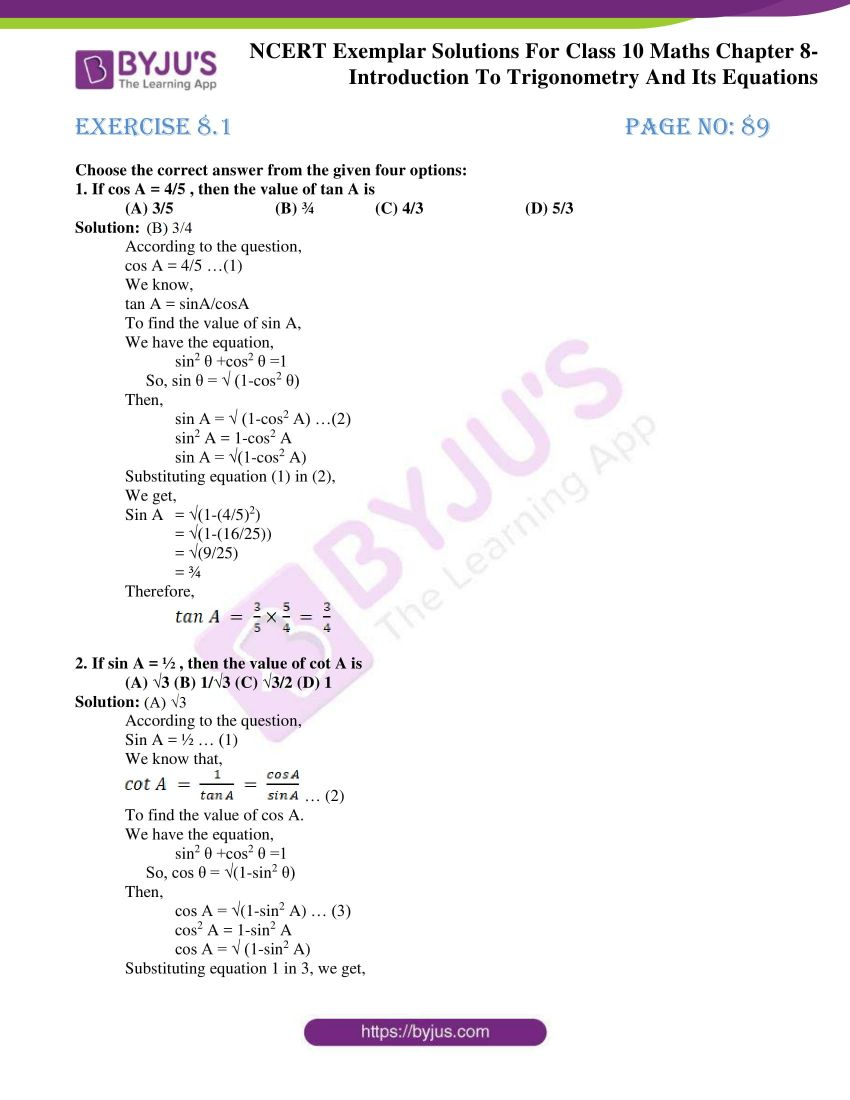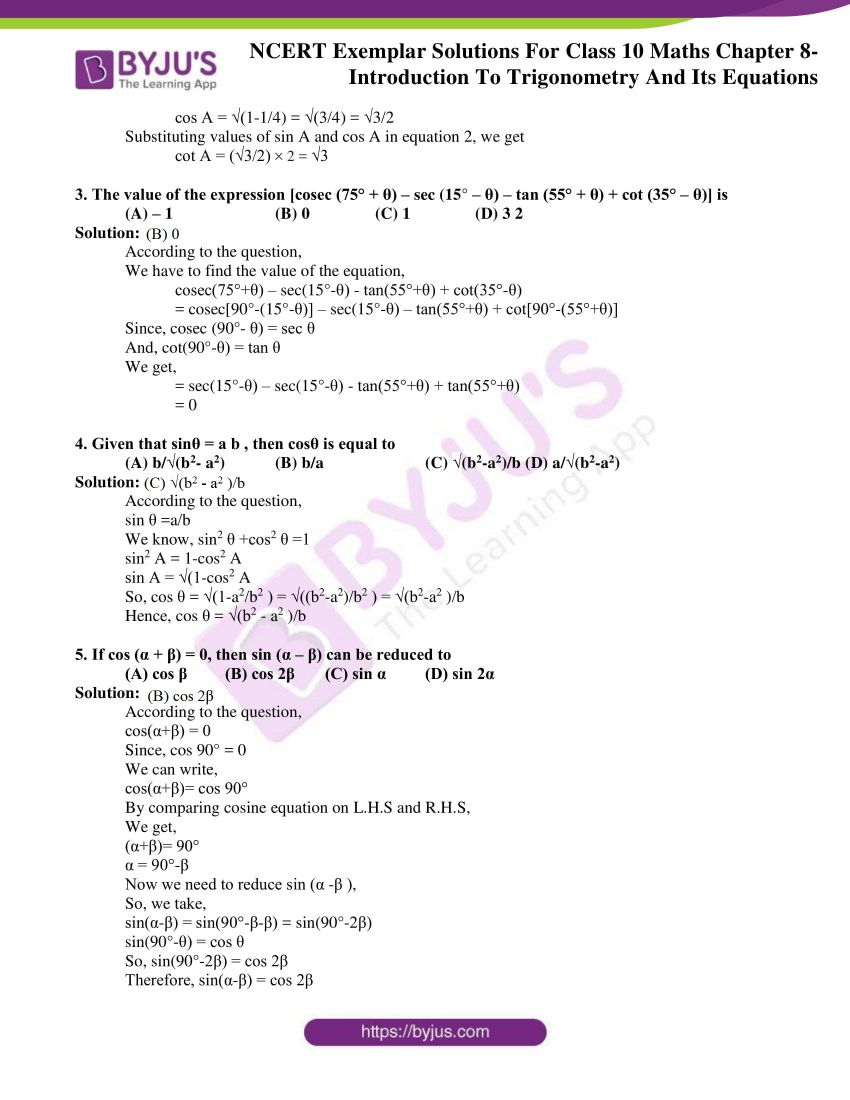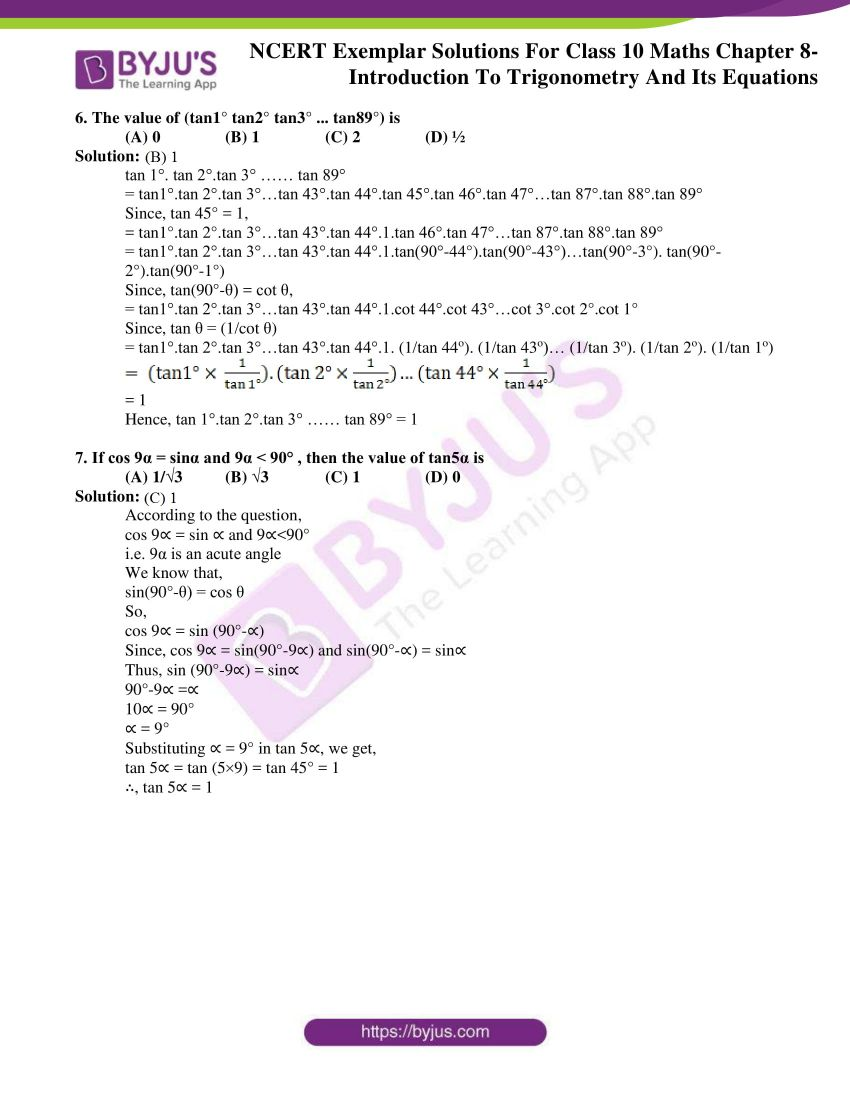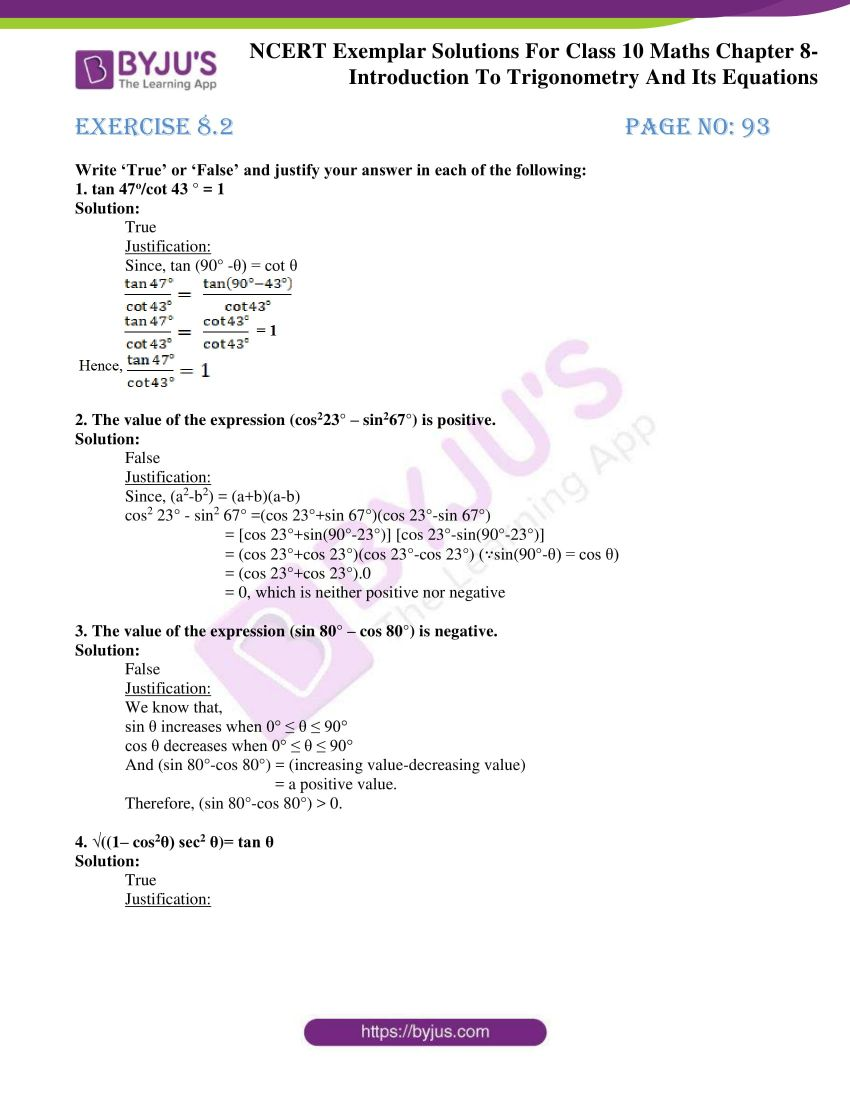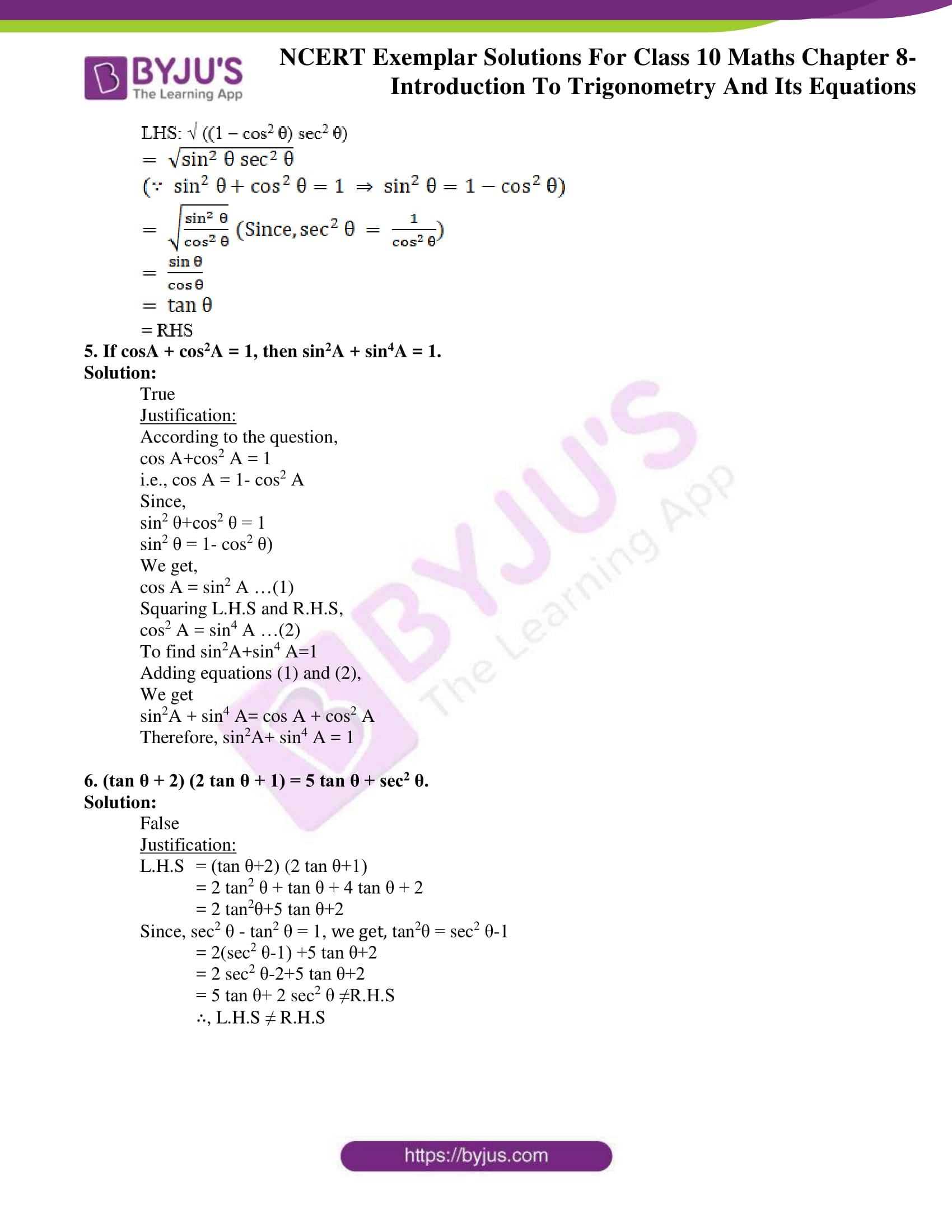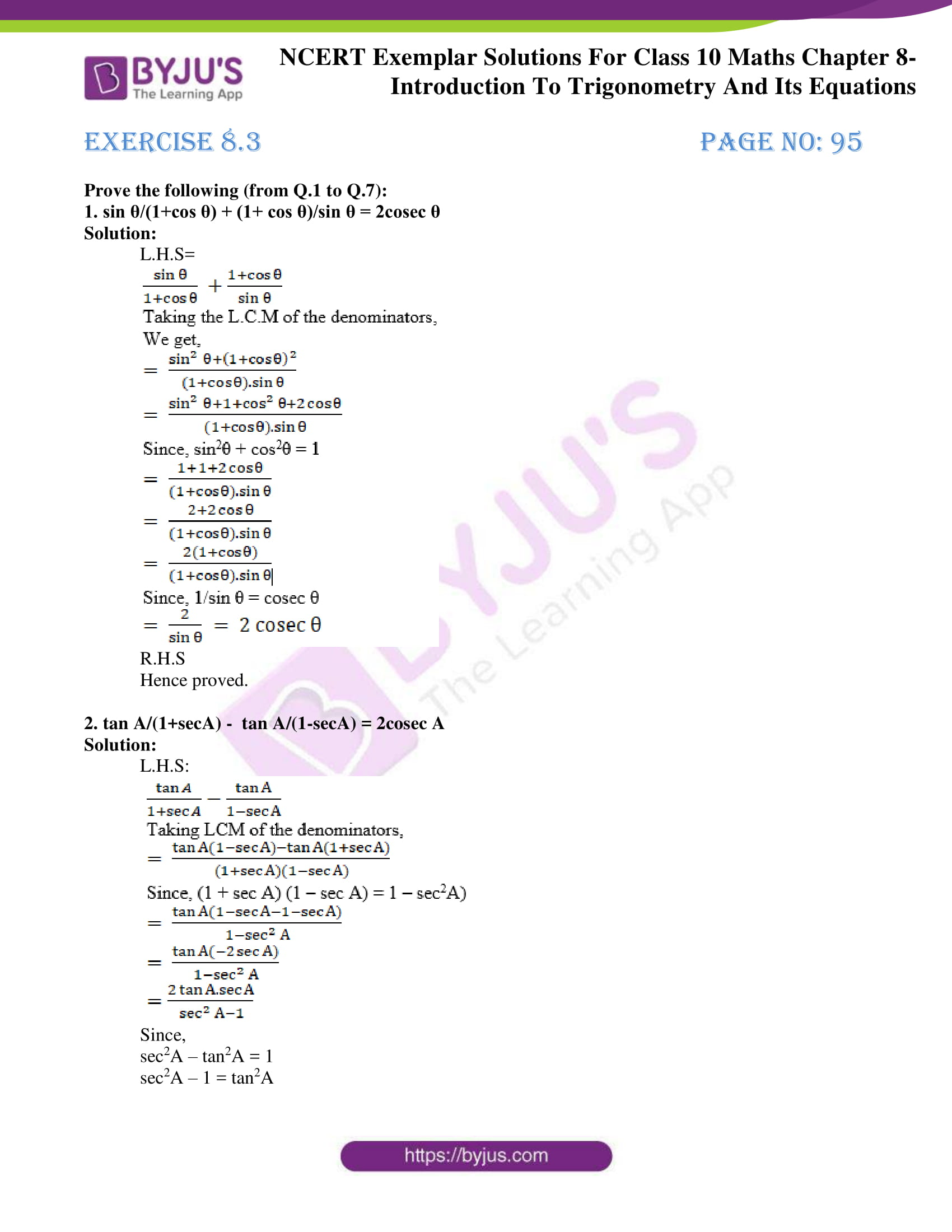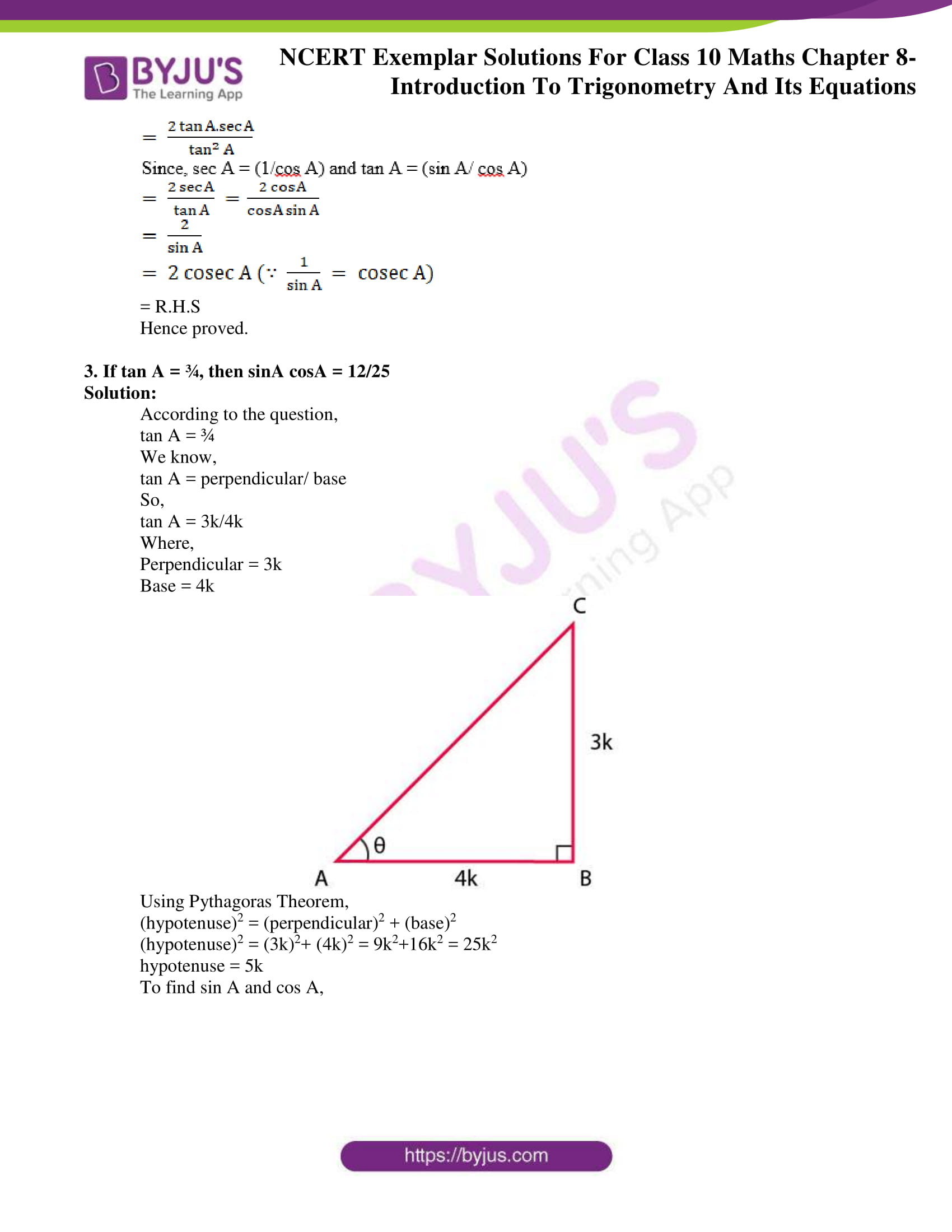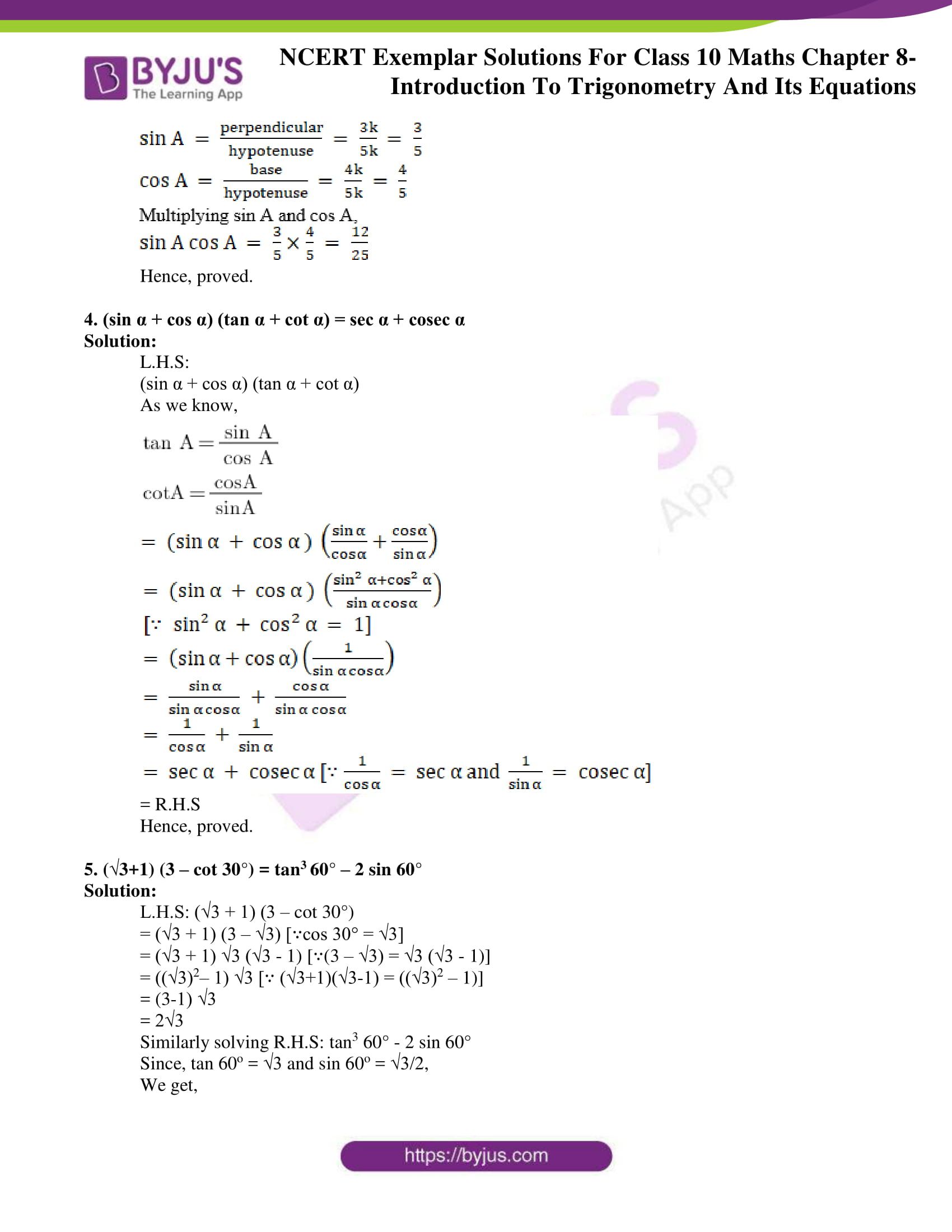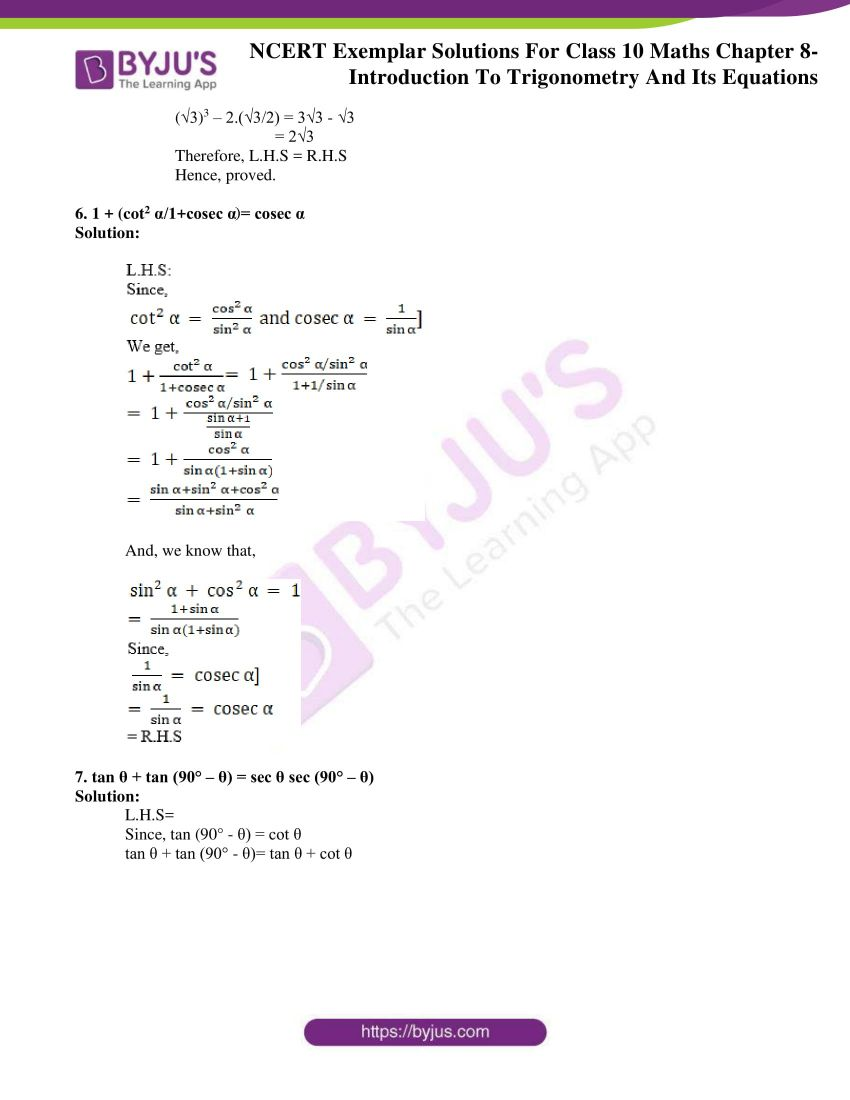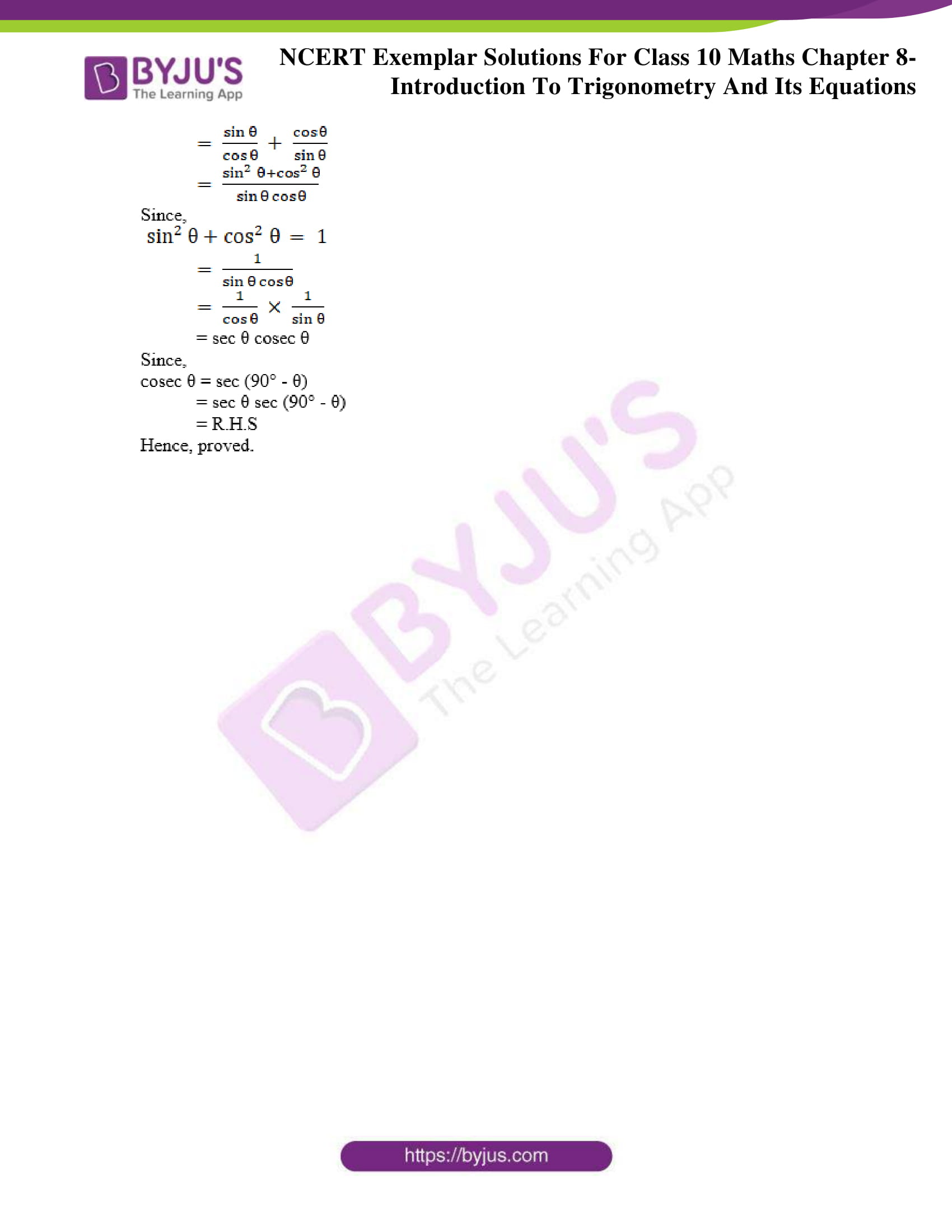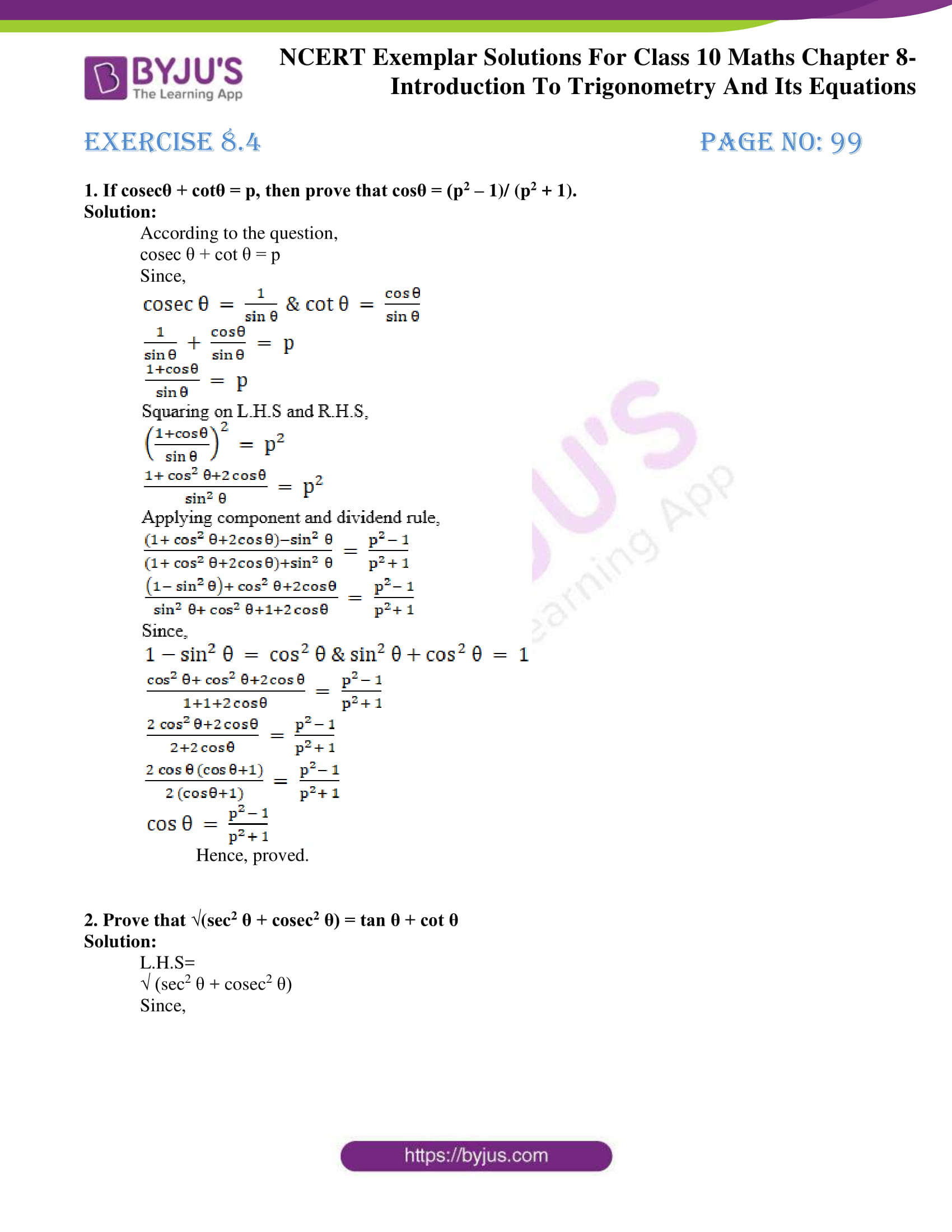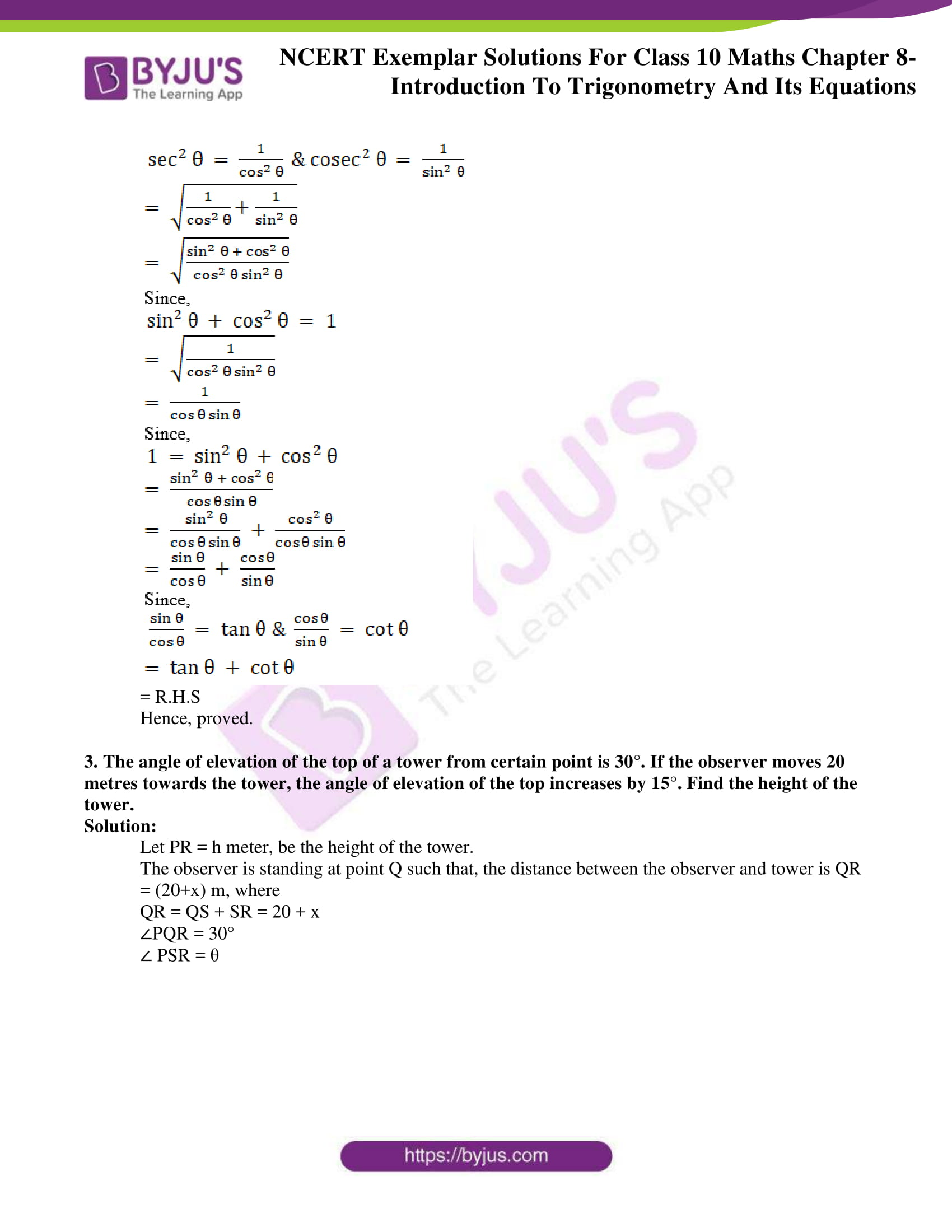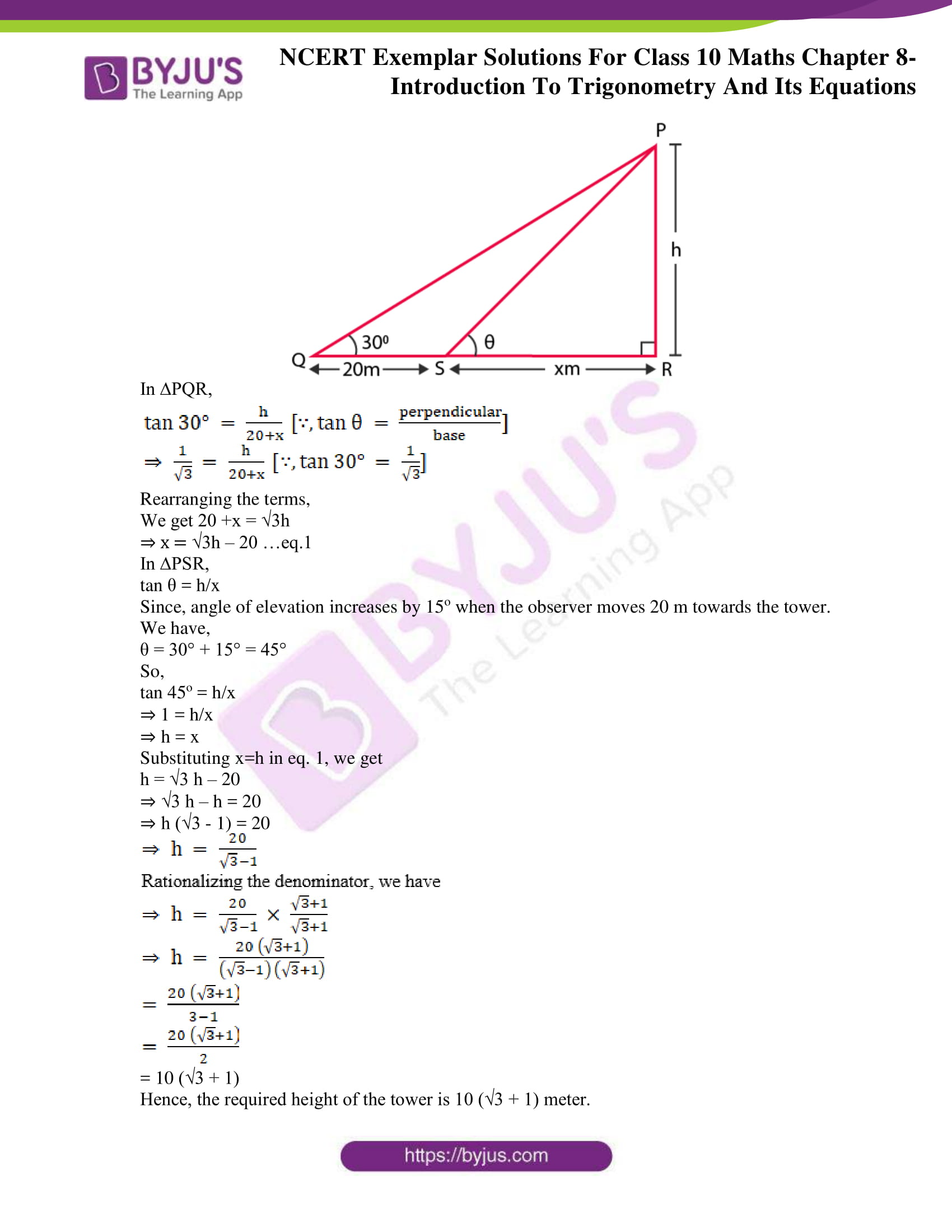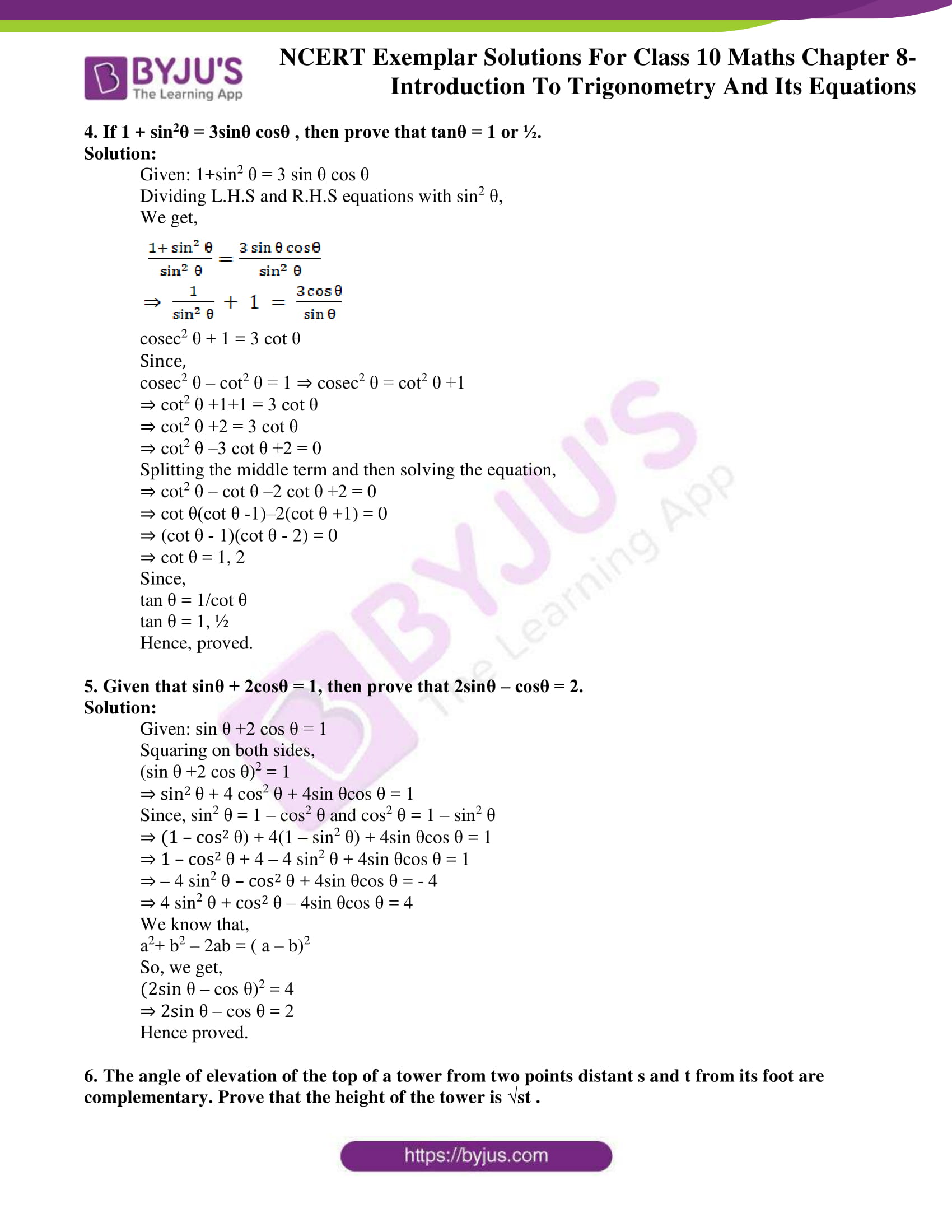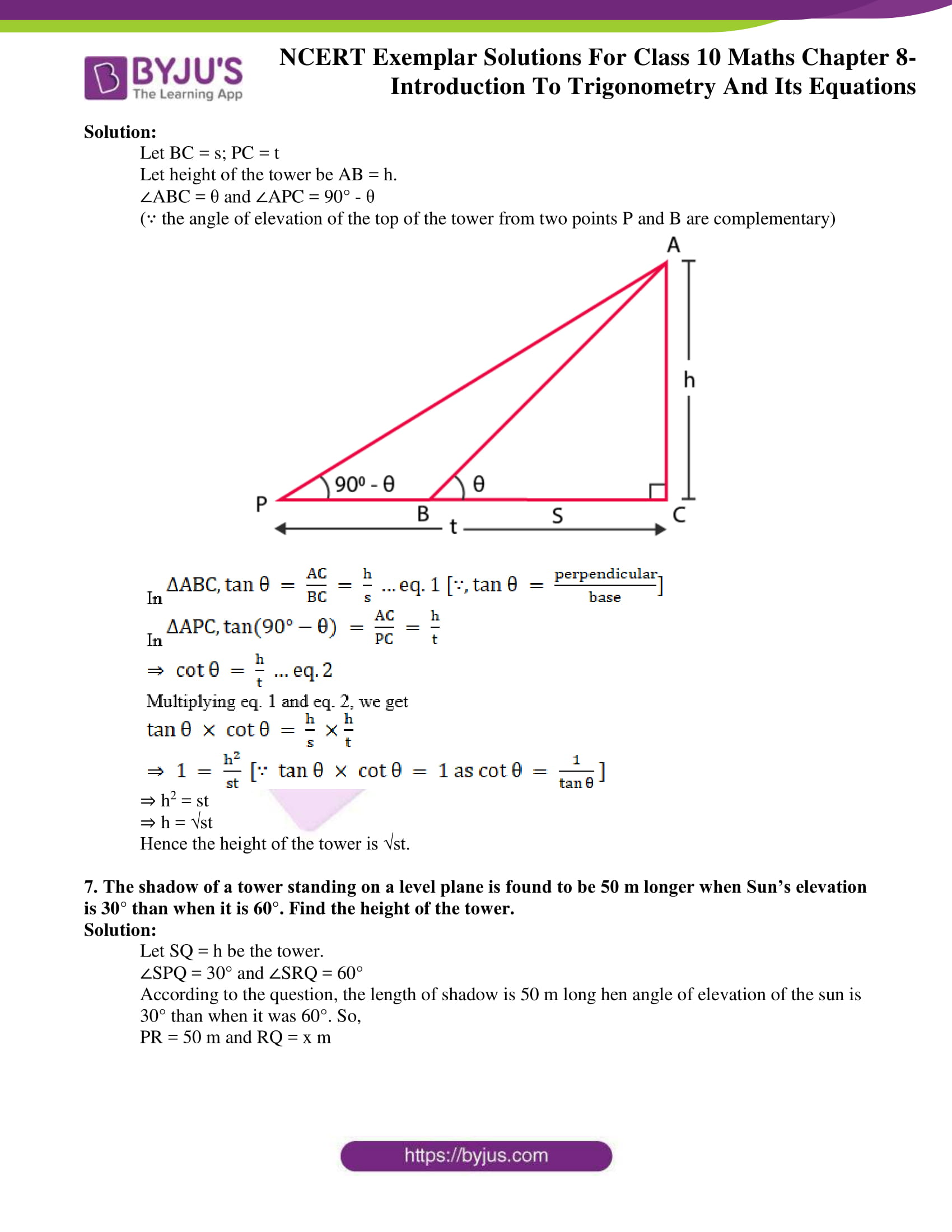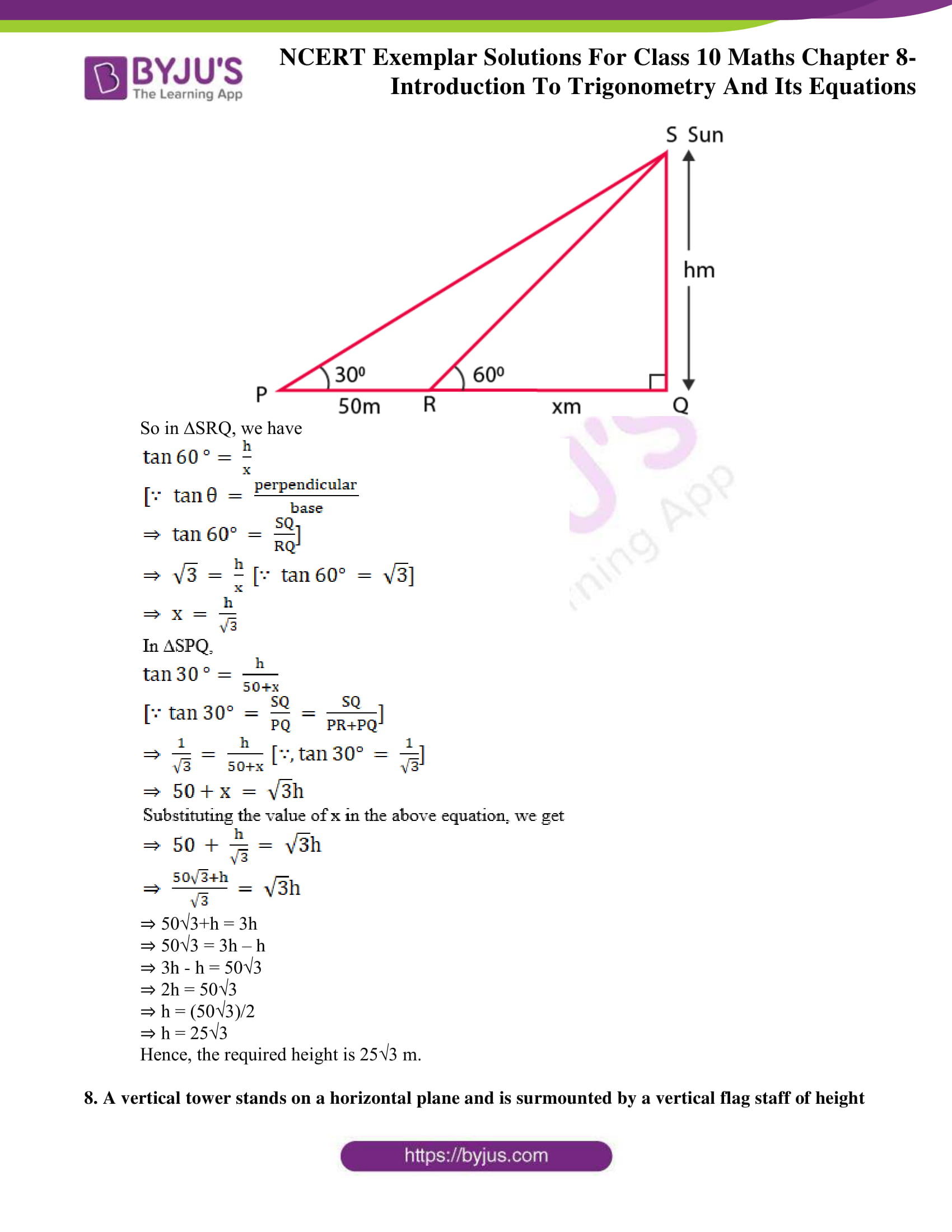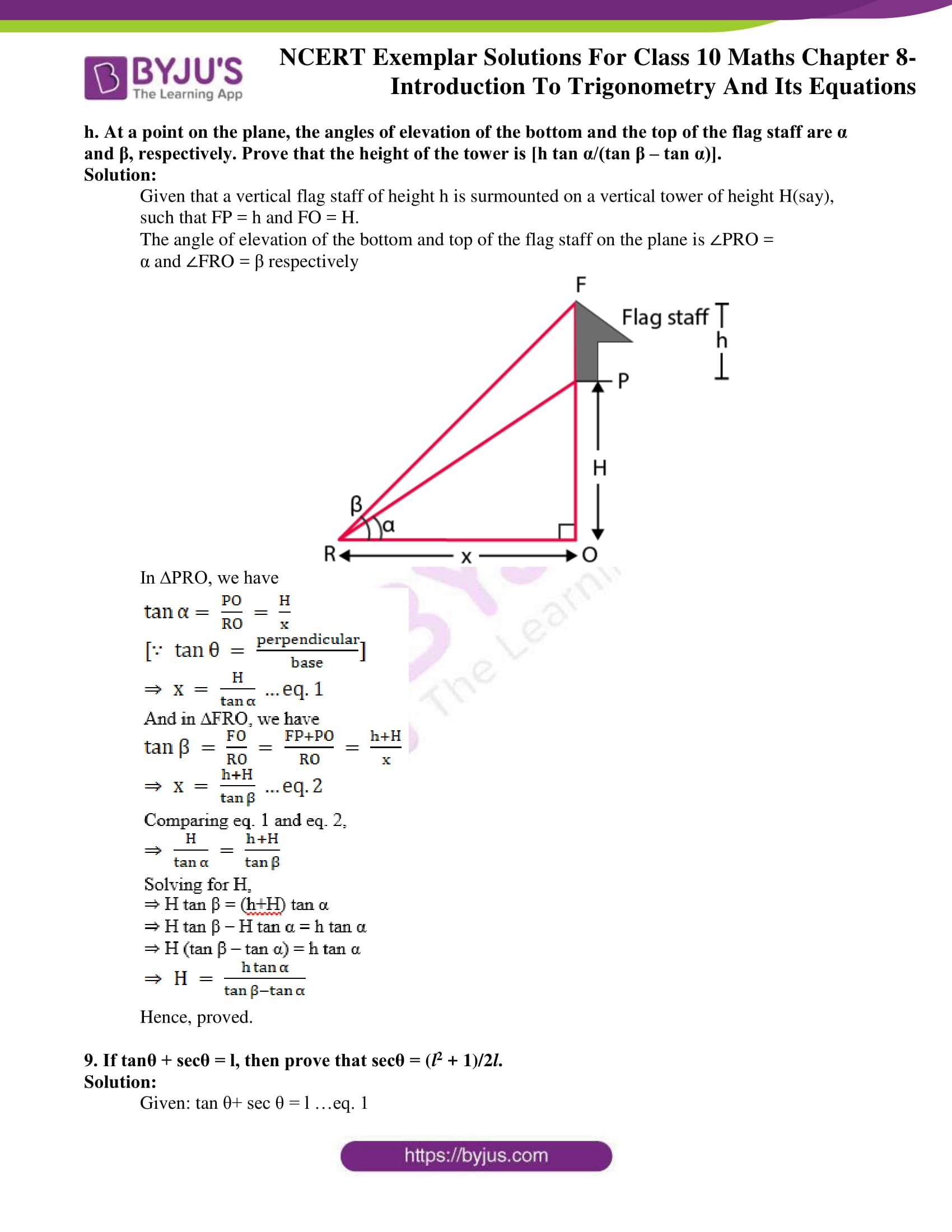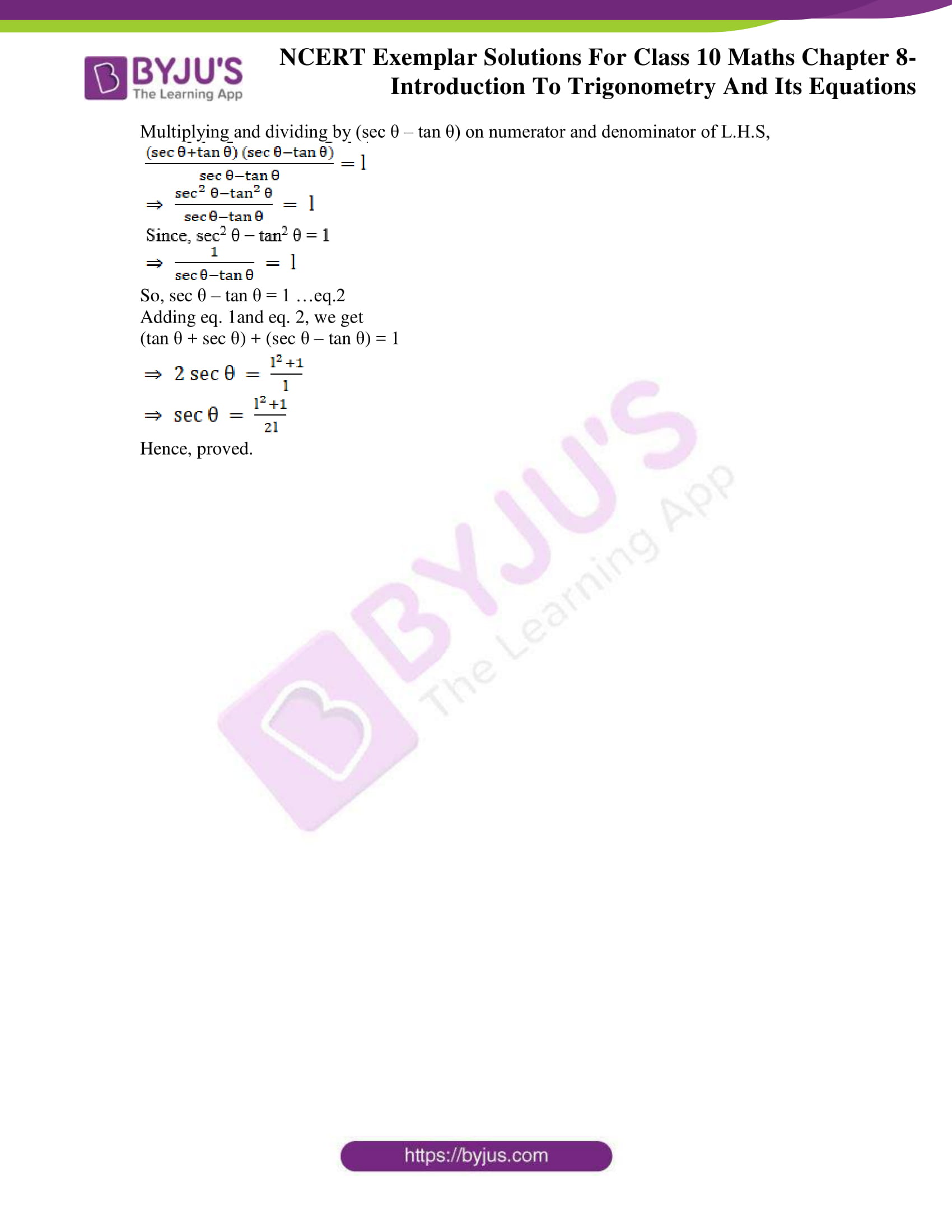## Exercise 8.1 Page No: 89

Choose the correct answer from the given four options:

1. If cos A = 4/5, then the value of tan A is

(A) 3/5 (B) ¾ (C) 4/3 (D) 5/3

Solution:

(B) 3/4

According to the question,

cos A = 4/5 …(1)

We know,

tan A = sinA/cosA

To find the value of sin A,

We have the equation,

sin2 θ +cos2 θ =1

So, sin θ = √ (1-cos2 θ)

Then,

sin A = √ (1-cos2 A) …(2)

sin2 A = 1-cos2 A

sin A = √(1-cos2 A)

Substituting equation (1) in (2),

We get,

Sin A = √(1-(4/5)2)

= √(1-(16/25))

= √(9/25)

= ¾

Therefore,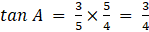2. If sin A = ½ , then the value of cot A is

(A) √3 (B) 1/√3 (C) √3/2 (D) 1

Solution:

(A) √3

According to the question,

Sin A = ½ … (1)

We know that,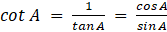… (2)

To find the value of cos A.

We have the equation,

sin2 θ +cos2 θ =1

So, cos θ = √(1-sin2 θ)

Then,

cos A = √(1-sin2 A) … (3)

cos2 A = 1-sin2 A

cos A = √ (1-sin2 A)

Substituting equation 1 in 3, we get,

cos A = √(1-1/4) = √(3/4) = √3/2

Substituting values of sin A and cos A in equation 2, we get

cot A = (√3/2) × 2 = √3

3. The value of the expression [cosec (75° + θ) – sec (15° – θ) – tan (55° + θ) + cot (35° – θ)] is

(A) – 1 (B) 0 (C) 1 (D) 3 2

Solution:

(B) 0

According to the question,

We have to find the value of the equation,

cosec(75°+θ) – sec(15°-θ) – tan(55°+θ) + cot(35°-θ)

= cosec[90°-(15°-θ)] – sec(15°-θ) – tan(55°+θ) + cot[90°-(55°+θ)]

Since, cosec (90°- θ) = sec θ

And, cot(90°-θ) = tan θ

We get,

= sec(15°-θ) – sec(15°-θ) – tan(55°+θ) + tan(55°+θ)

= 0

4. Given that sinθ = a b , then cosθ is equal to

(A) b/√(b2– a2) (B) b/a (C) √(b2-a2)/b (D) a/√(b2-a2)

Solution:

(C) √(b2 – a2)/b

According to the question,

sin θ =a/b

We know, sin2 θ +cos2 θ =1

sin2 A = 1-cos2 A

sin A = √(1-cos2 A

So, cos θ = √(1-a2/b2 ) = √((b2-a2)/b2 ) = √(b2-a2 )/b

Hence, cos θ = √(b2 – a2 )/b

5. If cos (α + β) = 0, then sin (α – β) can be reduced to

(A) cos β (B) cos 2β (C) sin α (D) sin 2α

Solution:

(B) cos 2β

According to the question,

cos(α+β) = 0

Since, cos 90° = 0

We can write,

cos(α+β)= cos 90°

By comparing cosine equation on L.H.S and R.H.S,

We get,

(α+β)= 90°

α = 90°-β

Now we need to reduce sin (α -β ),

So, we take,

sin(α-β) = sin(90°-β-β) = sin(90°-2β)

sin(90°-θ) = cos θ

So, sin(90°-2β) = cos 2β

Therefore, sin(α-β) = cos 2β

6. The value of (tan1° tan2° tan3° … tan89°) is

(A) 0 (B) 1 (C) 2 (D) ½

Solution:

(B) 1

tan 1°. tan 2°.tan 3° …… tan 89°

= tan1°.tan 2°.tan 3°…tan 43°.tan 44°.tan 45°.tan 46°.tan 47°…tan 87°.tan 88°.tan 89°

Since, tan 45° = 1,

= tan1°.tan 2°.tan 3°…tan 43°.tan 44°.1.tan 46°.tan 47°…tan 87°.tan 88°.tan 89°

= tan1°.tan 2°.tan 3°…tan 43°.tan 44°.1.tan(90°-44°).tan(90°-43°)…tan(90°-3°). tan(90°-2°).tan(90°-1°)

Since, tan(90°-θ) = cot θ,

= tan1°.tan 2°.tan 3°…tan 43°.tan 44°.1.cot 44°.cot 43°…cot 3°.cot 2°.cot 1°

Since, tan θ = (1/cot θ)

= tan1°.tan 2°.tan 3°…tan 43°.tan 44°.1. (1/tan 44o). (1/tan 43o)… (1/tan 3o). (1/tan 2o). (1/tan 1o)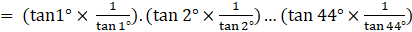= 1

Hence, tan 1°.tan 2°.tan 3° …… tan 89° = 1

7. If cos 9α = sinα and 9α < 90°, then the value of tan5α is

(A) 1/√3 (B) √3 (C) 1 (D) 0

Solution:

(C) 1

According to the question,

cos 9∝ = sin ∝ and 9∝<90°

i.e. 9α is an acute angle

We know that,

sin(90°-θ) = cos θ

So,

cos 9∝ = sin (90°-∝)

Since, cos 9∝ = sin(90°-9∝) and sin(90°-∝) = sin∝

Thus, sin (90°-9∝) = sin∝

90°-9∝ =∝

10∝ = 90°

∝ = 9°

Substituting ∝ = 9° in tan 5∝, we get,

tan 5∝ = tan (5×9) = tan 45° = 1

∴, tan 5∝ = 1

## Exercise 8.2 Page No: 93

Write ‘True’ or ‘False’ and justify your answer in each of the following:

1. tan 47o/cot 43 ° = 1

Solution:

True

Justification:

Since, tan (90° -θ) = cot θ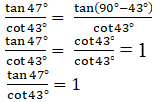2. The value of the expression (cos223° – sin267°) is positive.

Solution:

False

Justification:

Since, (a2-b2) = (a+b)(a-b)

cos2 23° – sin2 67° =(cos 23°+sin 67°)(cos 23°-sin 67°)

= [cos 23°+sin(90°-23°)] [cos 23°-sin(90°-23°)]

= (cos 23°+cos 23°)(cos 23°-cos 23°) (∵sin(90°-θ) = cos θ)

= (cos 23°+cos 23°).0

= 0, which is neither positive nor negative

3. The value of the expression (sin 80° – cos 80°) is negative.

Solution:

False

Justification:

We know that,

sin θ increases when 0° ≤ θ ≤ 90°

cos θ decreases when 0° ≤ θ ≤ 90°

And (sin 80°-cos 80°) = (increasing value-decreasing value)

= a positive value.

Therefore, (sin 80°-cos 80°) > 0.

4. √((1– cos2θ) sec2 θ)= tan θ

Solution:

True

Justification: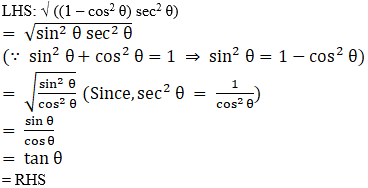5. If cosA + cos2A = 1, then sin2A + sin4A = 1.

Solution:

True

Justification:

According to the question,

cos A+cos2 A = 1

i.e., cos A = 1- cos2 A

Since,

sin2 θ+cos2 θ = 1

sin2 θ = 1- cos2 θ)

We get,

cos A = sin2 A …(1)

Squaring L.H.S and R.H.S,

cos2 A = sin4 A …(2)

To find sin2A+sin4 A=1

We get

sin2A + sin4 A= cos A + cos2 A

Therefore, sin2A+ sin4 A = 1

6. (tan θ + 2) (2 tan θ + 1) = 5 tan θ + sec2 θ.

Solution:

False

Justification:

L.H.S = (tan θ+2) (2 tan θ+1)

= 2 tan2 θ + tan θ + 4 tan θ + 2

= 2 tan2θ+5 tan θ+2

Since, sec2 θ – tan2 θ = 1, we get, tan2θ = sec2 θ-1

= 2(sec2 θ-1) +5 tan θ+2

= 2 sec2 θ-2+5 tan θ+2

= 5 tan θ+ 2 sec2 θ ≠R.H.S

∴, L.H.S ≠ R.H.S

## Exercise 8.3 Page No: 95

Prove the following (from Q.1 to Q.7):

1. sin θ/(1+cos θ) + (1+ cos θ)/sin θ = 2cosec θ

Solution:

L.H.S=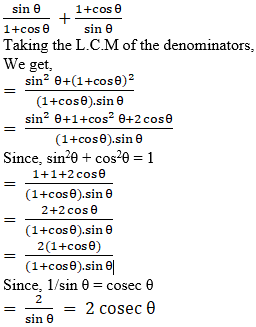R.H.S

Hence proved.

2. tan A/(1+secA) – tan A/(1-secA) = 2cosec A

Solution:

L.H.S: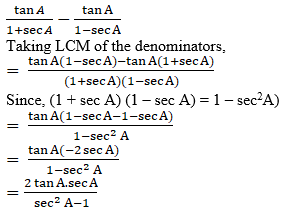Since,

sec2A – tan2A = 1

sec2A – 1 = tan2A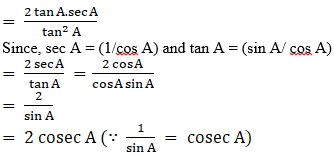= R.H.S

Hence proved.

3. If tan A = ¾, then sinA cosA = 12/25

Solution:

According to the question,

tan A = ¾

We know,

tan A = perpendicular/ base

So,

tan A = 3k/4k

Where,

Perpendicular = 3k

Base = 4k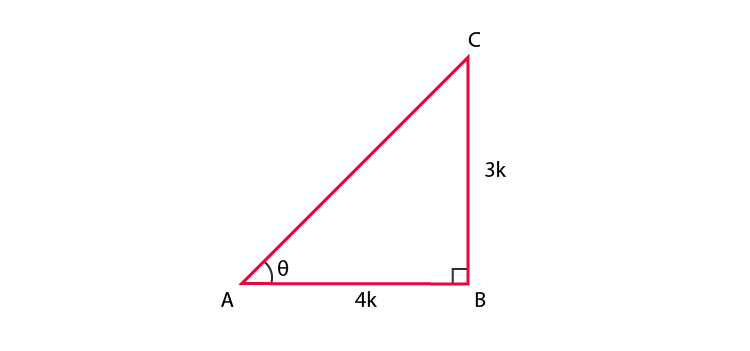Using Pythagoras Theorem,

(hypotenuse)2 = (perpendicular)2 + (base)2

(hypotenuse)2 = (3k)2+ (4k)2 = 9k2+16k2 = 25k2

hypotenuse = 5k

To find sin A and cos A,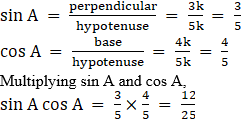Hence, proved.

4. (sin α + cos α) (tan α + cot α) = sec α + cosec α

Solution:

L.H.S:

(sin α + cos α) (tan α + cot α)

As we know,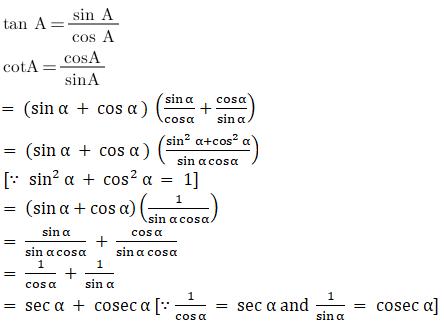= R.H.S

Hence, proved.

5. (√3+1) (3 – cot 30°) = tan3 60° – 2 sin 60°

Solution:

L.H.S: (√3 + 1) (3 – cot 30°)

= (√3 + 1) (3 – √3) [∵cos 30° = √3]

= (√3 + 1) √3 (√3 – 1) [∵(3 – √3) = √3 (√3 – 1)]

= ((√3)2– 1) √3 [∵ (√3+1)(√3-1) = ((√3)2 – 1)]

= (3-1) √3

= 2√3

Similarly solving R.H.S: tan3 60° – 2 sin 60°

Since, tan 60o = √3 and sin 60o = √3/2,

We get,

(√3)3 – 2.(√3/2) = 3√3 – √3

= 2√3

Therefore, L.H.S = R.H.S

Hence, proved.

6. 1 + (cot2 α/1+cosec α) = cosec α

Solution: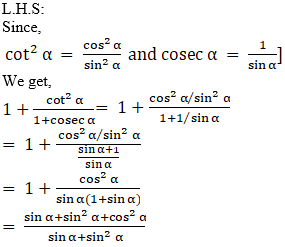And, we know that,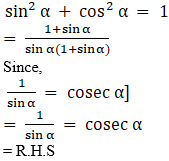7. tan θ + tan (90° – θ) = sec θ sec (90° – θ)

Solution:

L.H.S=

Since, tan (90° – θ) = cot θ

tan θ + tan (90° – θ)= tan θ + cot θ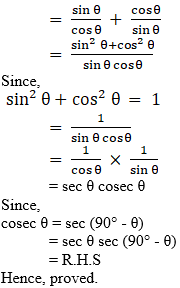## Exercise 8.4 Page No: 99

1. If cosecθ + cotθ = p, then prove that cosθ = (p2 – 1)/ (p2 + 1).

Solution:

According to the question,

cosec θ + cot θ = p

Since,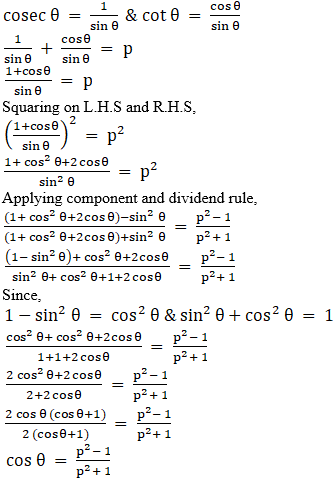Hence, proved.

2. Prove that √(sec2 θ + cosec2 θ) = tan θ + cot θ

Solution:

L.H.S=

√ (sec2 θ + cosec2 θ)

Since,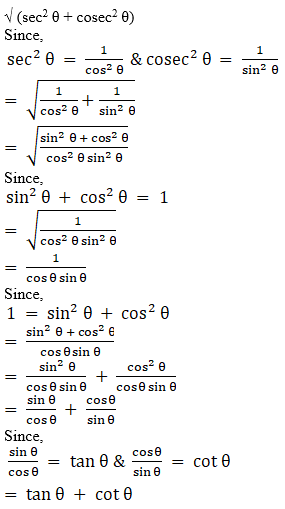= R.H.S

Hence, proved.

3. The angle of elevation of the top of a tower from certain point is 30°. If the observer moves 20 metres towards the tower, the angle of elevation of the top increases by 15°. Find the height of the tower.

Solution:

Let PR = h meter, be the height of the tower.

The observer is standing at point Q such that, the distance between the observer and tower is QR = (20+x) m, where

QR = QS + SR = 20 + x

∠PQR = 30°

∠ PSR = θ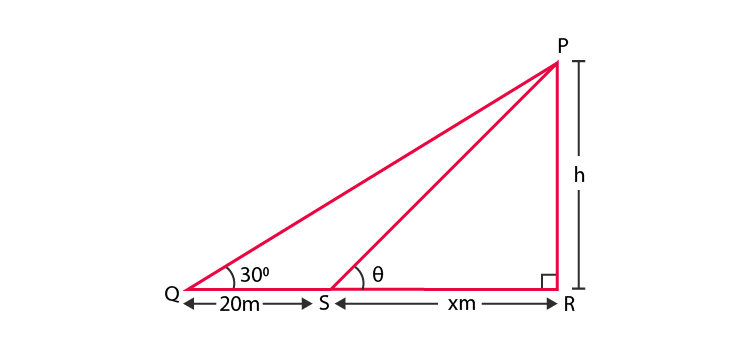In ∆PQR,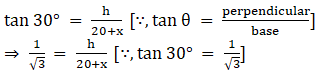Rearranging the terms,

We get 20 +x = √3h

⇒ x = √3h – 20 …eq.1

In ∆PSR,

tan θ = h/x

Since, angle of elevation increases by 15o when the observer moves 20 m towards the tower.

We have,

θ = 30° + 15° = 45°

So,

tan 45o = h/x

⇒ 1 = h/x

⇒ h = x

Substituting x=h in eq. 1, we get

h = √3 h – 20

⇒ √3 h – h = 20

⇒ h (√3 – 1) = 20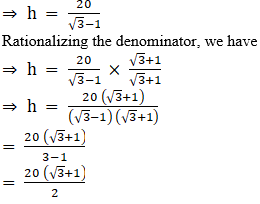= 10 (√3 + 1)

Hence, the required height of the tower is 10 (√3 + 1) meter.

4. If 1 + sin2θ = 3sinθ cosθ , then prove that tanθ = 1 or ½.

Solution:

Given: 1+sin2 θ = 3 sin θ cos θ

Dividing L.H.S and R.H.S equations with sin2 θ,

We get,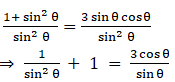cosec2 θ + 1 = 3 cot θ

Since,

cosec2 θ – cot2 θ = 1 ⇒ cosec2 θ = cot2 θ +1

⇒ cot2 θ +1+1 = 3 cot θ

⇒ cot2 θ +2 = 3 cot θ

⇒ cot2 θ –3 cot θ +2 = 0

Splitting the middle term and then solving the equation,

⇒ cot2 θ – cot θ –2 cot θ +2 = 0

⇒ cot θ(cot θ -1)–2(cot θ +1) = 0

⇒ (cot θ – 1)(cot θ – 2) = 0

⇒ cot θ = 1, 2

Since,

tan θ = 1/cot θ

tan θ = 1, ½

Hence, proved.

5. Given that sinθ + 2cosθ = 1, then prove that 2sinθ – cosθ = 2.

Solution:

Given: sin θ +2 cos θ = 1

Squaring on both sides,

(sin θ +2 cos θ)2 = 1

⇒ sin2 θ + 4 cos2 θ + 4sin θcos θ = 1

Since, sin2 θ = 1 – cos2 θ and cos2 θ = 1 – sin2 θ

⇒ (1 – cos2 θ) + 4(1 – sin2 θ) + 4sin θcos θ = 1

⇒ 1 – cos2 θ + 4 – 4 sin2 θ + 4sin θcos θ = 1

⇒ – 4 sin2 θ – cos2 θ + 4sin θcos θ = – 4

⇒ 4 sin2 θ + cos2 θ – 4sin θcos θ = 4

We know that,

a2+ b2 – 2ab = ( a – b)2

So, we get,

(2sin θ – cos θ)2 = 4

⇒ 2sin θ – cos θ = 2

Hence proved.

6. The angle of elevation of the top of a tower from two points distant s and t from its foot are complementary. Prove that the height of the tower is √st .

Solution:

Let BC = s; PC = t

Let height of the tower be AB = h.

∠ABC = θ and ∠APC = 90° – θ

(∵ the angle of elevation of the top of the tower from two points P and B are complementary)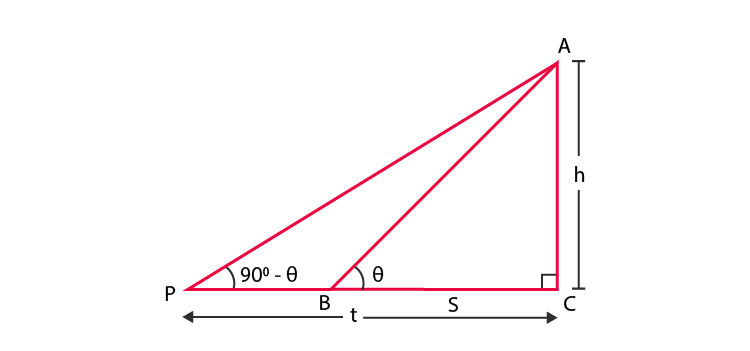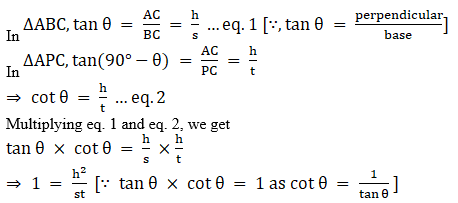⇒ h2 = st

⇒ h = √st

Hence the height of the tower is √st.

7. The shadow of a tower standing on a level plane is found to be 50 m longer when Sun’s elevation is 30° than when it is 60°. Find the height of the tower.

Solution:

Let SQ = h be the tower.

∠SPQ = 30° and ∠SRQ = 60°

According to the question, the length of shadow is 50 m long hen angle of elevation of the sun is 30° than when it was 60°. So,

PR = 50 m and RQ = x m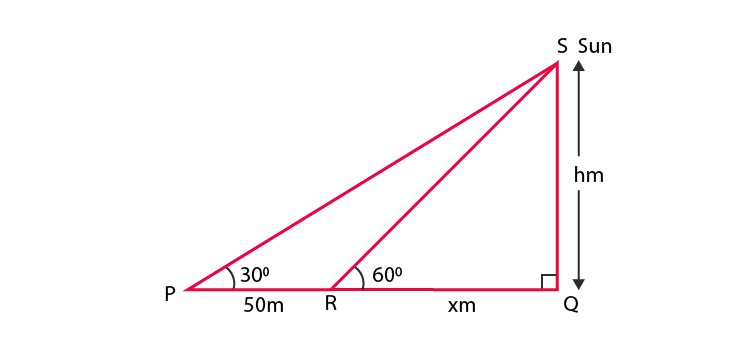So in ∆SRQ, we have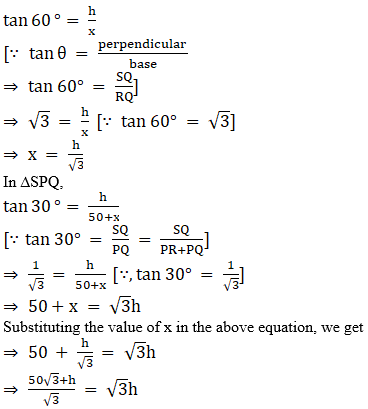⇒ 50√3+h = 3h

⇒ 50√3 = 3h – h

⇒ 3h – h = 50√3

⇒ 2h = 50√3

⇒ h = (50√3)/2

⇒ h = 25√3

Hence, the required height is 25√3 m.

8. A vertical tower stands on a horizontal plane and is surmounted by a vertical flag staff of height h. At a point on the plane, the angles of elevation of the bottom and the top of the flag staff are α and β, respectively. Prove that the height of the tower is [h tan α/(tan β – tan α)].

Solution:

Given that a vertical flag staff of height h is surmounted on a vertical tower of height H(say),

such that FP = h and FO = H.

The angle of elevation of the bottom and top of the flag staff on the plane is ∠PRO = α and ∠FRO = β respectively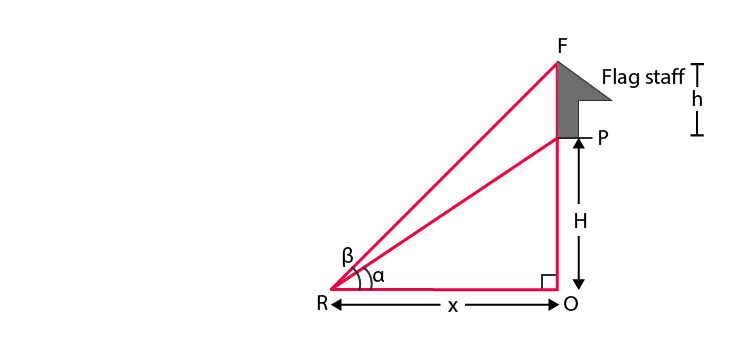In ∆PRO, we have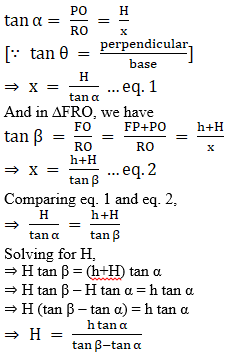Hence, proved.

9. If tanθ + secθ = l, then prove that secθ = (l2 + 1)/2l.

Solution:

Given: tan θ+ sec θ = l …eq. 1

Multiplying and dividing by (sec θ – tan θ) on numerator and denominator of L.H.S,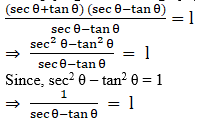So, sec θ – tan θ = 1 …eq.2

Adding eq. 1and eq. 2, we get

(tan θ + sec θ) + (sec θ – tan θ) = 1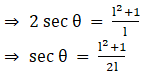Hence, proved.

Class 10 students are advised to solve sample papers and previous year question papers to have an idea of the type of questions asked from chapter Trigonometry. Also find our online reading materials such as notes, exemplar books, 10th Class Maths NCERT Solutions and question papers in downloadable PDF format to have a quick revision before the exam.

Download BYJU’S app to get personalized video lessons explaining different types of Maths topics and experience a new method of learning to understand the concepts in an easy way.

## Frequently Asked Questions on NCERT Exemplar Solutions for Class 10 Maths Chapter 8

### Explain the concept of trigonometric ratios covered in Chapter 8 of NCERT Exemplar Solutions for Class 10 Maths.

Trigonometric Ratios are defined as the values of all the trigonometric functions based on the value of the ratio of sides in a right-angled triangle. The ratios of sides of a right-angled triangle with respect to any of its acute angles are known as the trigonometric ratios of that particular angle.
The three sides of the right triangle are:
1. Hypotenuse (the longest side)
2. Perpendicular (opposite side to the angle)
3. Base (Adjacent side to the angle)
The introduction of this chapter has definitions of terms which are important for the exams. Students can now study and be updated about the latest syllabus of the CBSE board using the NCERT Exemplar Solutions which are available in PDF format.

### Will the NCERT Exemplar Solutions for Class 10 Maths Chapter 8 help students to understand the concepts which are important from the exam perspective?

In order to understand the expansion procedure, students can refer to the examples which are present in the NCERT textbook before solving the exercise wise problems. Each problem in the solutions is solved in a stepwise manner to help students in understanding the concepts in a better way. By using the solutions PDF, students will be well versed with the method of solving these equations and score well in the exam.

### What are the topics covered in the NCERT Exemplar Solutions for Class 10 Maths Chapter 8?

In this chapter, they will learn solving problems based on trigonometric ratios for specific and complementary angles and establishing identities for the trigonometric ratios. This chapter covers the following topics of trigonometry given below:
1. Finding the trigonometric ratios of the angle
2. Finding the trigonometric ratios of some specific angles such as 30°, 45°, 60°, 90° and 0°
3. Trigonometric ratios of complementary angles, like sin(90° – A)
4. Proofs based on Trigonometric Identities